06 103 624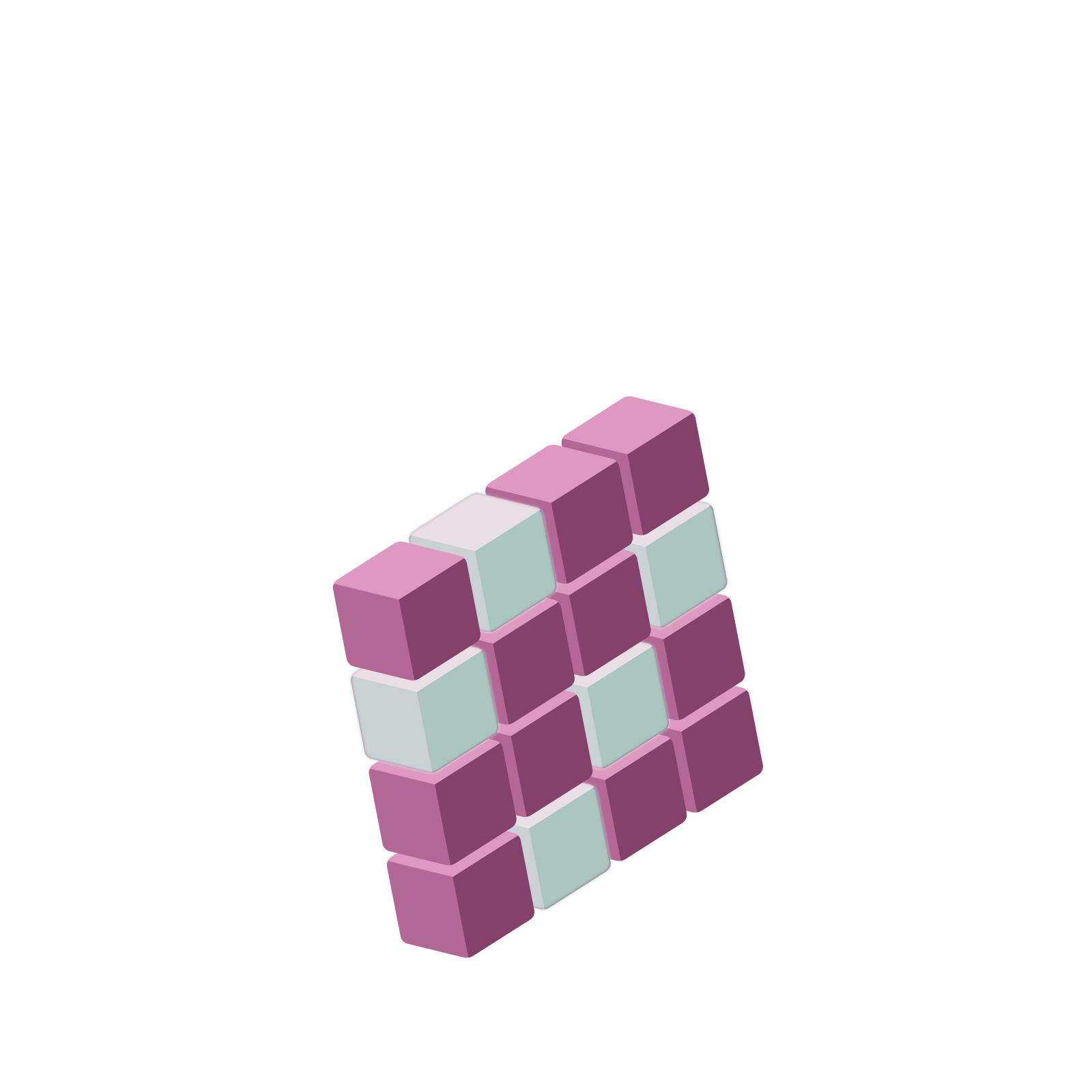# Station  10BA Troubling Number our Usual Mathematics RejectsThe number $0.9999\ldots$ (if you choose to believe it is a one) has infinitely many $9$s to the right of the decimal point. What if we consider the “number” with infinitely many $9$s to the left of the decimal point instead?

$\ldots9999$

This is a number that ends with nine. Actually it ends with ninety-nine. Actually it ends with nine-hundred-and-ninety-nine. And so on.Let’s apply our algebraic argument to see what value it must have.

STEP 1: Give the quantity a name.

We’ll call it $A$ for Allistaire.

$A = \ldots9999$.

STEP 2: Multiply by ten

We obtain

$10A = \ldots99990$.

STEP 3: Subtract

We see that $A$ and $10A$ differ by nine (it is only their final digits that differ). Looking at $A-10A$ we get

$-9A=9$

giving

$A=-1$.

That is, our mathematics establish that

$\ldots9999 = -1$.

Apparently, if we pulled out a calculator and computed the sum $9+90+900+9000+\cdots$ the calculator will show at the end of time the answer $-1$!

Do you believe that?

Putting it another way: On a number line, do you believe that the numbers $9$, $99$, $999$, …. are marching closer and closer to the number $-1$?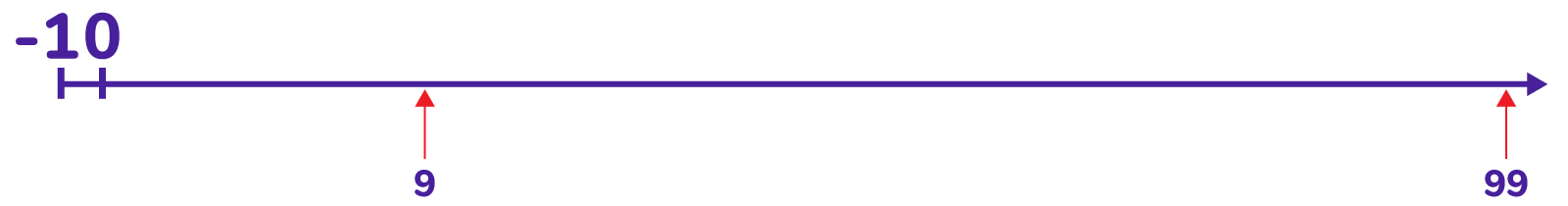Challenge: Let’s make matters worse! Consider the “number” with infinitely many $9$s both to the left and to the right of the decimal point: $\ldots9999.9999\ldots$. Use the same algebraic argument to show that this equals zero. (And this makes sense, because $\ldots9999.9999\ldots = \ldots9999+ .9999\ldots = -1 + 1 = 0$.)

It is hard to believe that $\ldots9999$ is a meaningful number and, moreover, it has the value $-1$, at least in our usual way of think about arithmetic. But remember, all we proved here is that IF we choose to say that $\ldots9999$ is a meaningful number, then it has value $-1$. It is up to us to decide whether or not it is meaningful quantity in the first place. Most people say it is not and stop there and that is fine.

But this begs the question:

Is there an UNUSUAL system of arithmetic for which $\ldots9999$ is meaningful (for which it has value $-1$)?

Challenge: One might be able to argue that $\ldots9999$ does behave like $-1$ in ordinary arithmetic to some degree. For example, consider performing the (very) long addition shown. Do you see the answer zero results?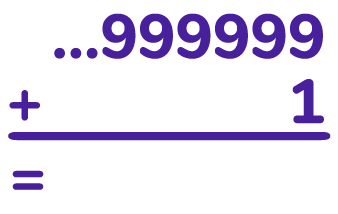If you prefer, imagine what happens if you add one dot to this loaded $1 \leftarrow 10$ machine.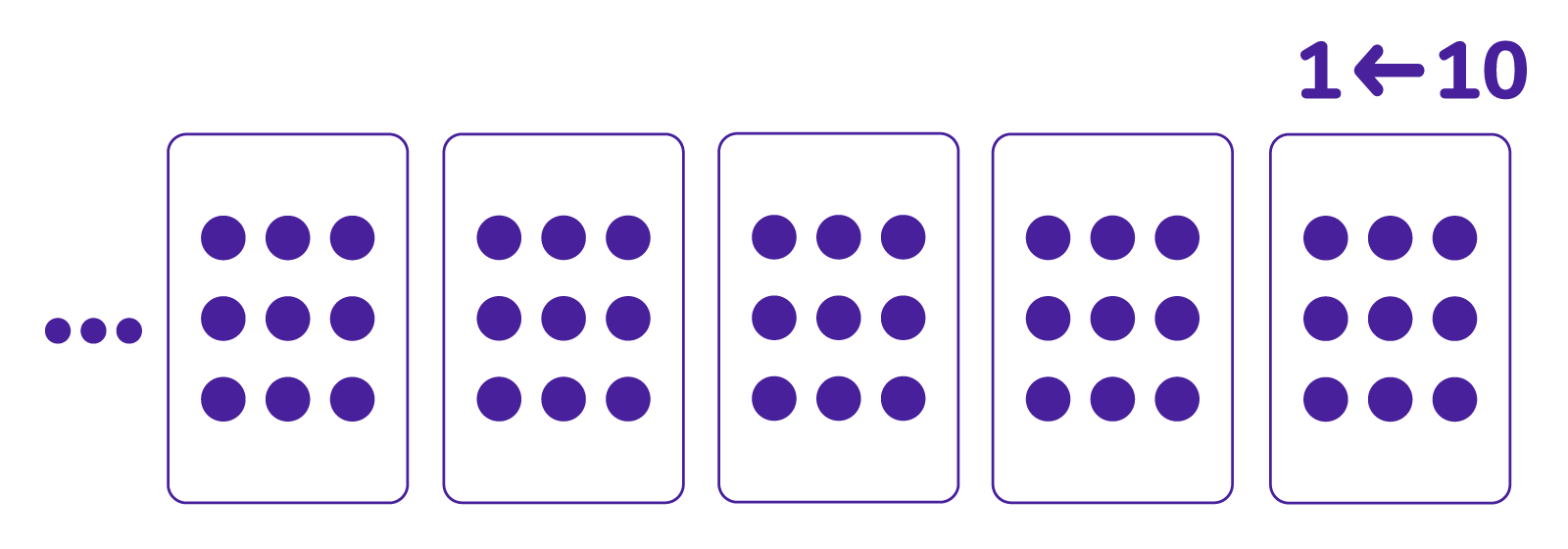Challenge: Try this (very) long multiplication problem. Do you see that $\ldots66667$ is behaving like the fraction $\dfrac{1}{3}$?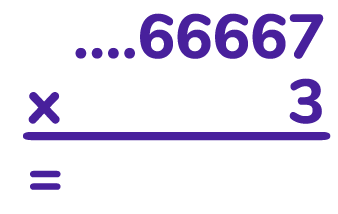If you prefer, imagine what happens if you triple the count of dots in each of these boxes.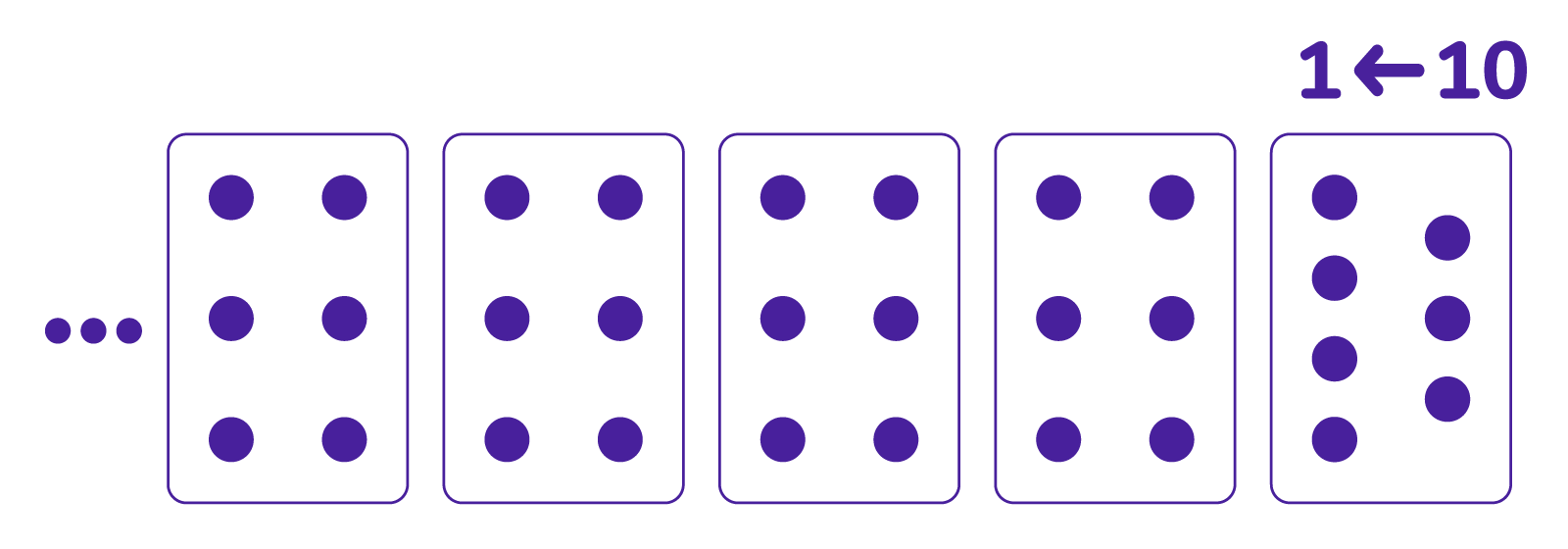Extra: What “number” behaves like $\dfrac{2}{3}$?